GeeksforGeeks App
Open AppBrowser
Continue

## Related Articles

• RD Sharma Class 9 Solutions

# Class 9 RD Sharma Solutions – Chapter 9 Triangles and its Angles- Exercise 9.2

### Question 1: The exterior angles, obtained on producing the base of a triangle both ways are 104° and 136°. Find all the angles of the triangle.

Solution: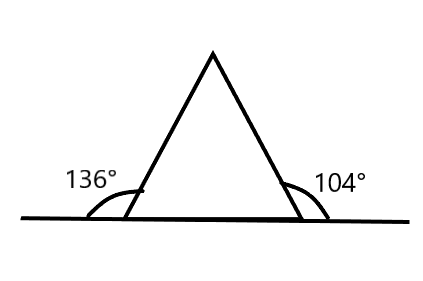Theorems Used: The exterior angle theorem states that the measure of each exterior angle of a triangle is equal to the sum of the opposite and non-adjacent interior angles. (Exterior Angle Theorem)

∠ACD = ∠ABC + ∠BAC [Exterior Angle Theorem]

Find ∠ABC:

∠ABC + ∠ABE = 180° [Linear pair]

∠ABC + 136° = 180°

∠ABC = 44°

Find ∠ACB:

∠ACB + ∠ACD = 180° [Linear pair]

∠ACB + 1040 = 180°

∠ACB = 76°

Now,

Sum of all angles of a triangle = 180°

∠A + 44° + 76° = 180°

∠A = 180° − 44°−76°

∠ A = 60°

Angles of the triangle are ∠ A = 60°, ∠B = 44° and ∠C = 76° (ans)

### Question 2: In an △ABC, the internal bisectors of ∠B and ∠C meet at P and the external bisectors of ∠B and ∠C meet at Q. Prove that ∠BPC + ∠BQC = 180°.

Solution:

In △ABC,

BP and CP are an internal bisector of ∠B and ∠C respectively

=> External ∠B = 180° – ∠B

BQ and CQ are an external bisector of ∠B and ∠C respectively.

=> External ∠C = 180° – ∠C

In triangle BPC,

∠BPC + 1/2∠B + 1/2∠C = 180°

∠BPC = 180° – (∠B + ∠C) …. (1)

In triangle BQC,

∠BQC + 1/2(180° – ∠B) + 1/2(180° – ∠C) = 180°

∠BQC + 180° – (∠B + ∠C) = 180°

∠BPC + ∠BQC = 180° [Using (1)] (Proved)

### Question 3: In figure, the sides BC, CA and AB of a △ABC have been produced to D, E and F respectively. If ∠ACD = 105° and ∠EAF = 45°, find all the angles of the △ABC.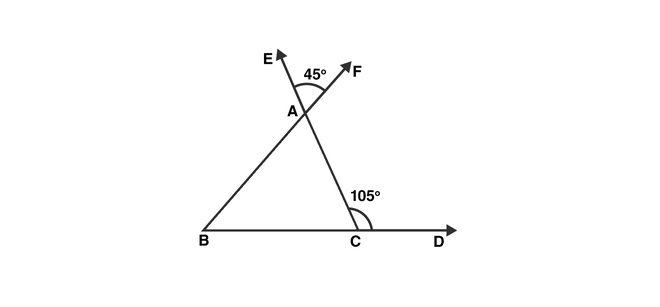Solution:

Theorems Used:

(i) The exterior angle theorem states that the measure of each exterior angle of a triangle is equal to the sum of the opposite and non-adjacent interior angles. (Exterior Angle Theorem)

(ii) Sum of a linear angle pair is 180°

(iii)Vertically opposite angles are equal.

∠BAC = ∠EAF = 45° [Vertically opposite angles]

∠ACD = 180° – 105° = 75° [Linear pair]

∠ABC = 105° – 45° = 60° [Exterior angle property]

### (i)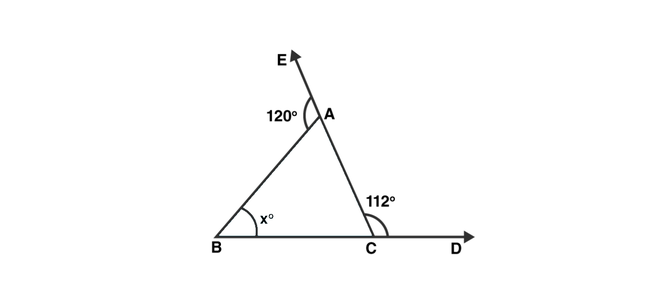Solution:

∠BAC = 180° – 120° = 60° [Linear pair]

∠ACB = 180° – 112° = 68° [Linear pair]

Sum of all angles of a triangle = 1800

x = 180° − ∠BAC − ∠ACB

= 180° − 60° − 68° = 52° (ans)

### (ii)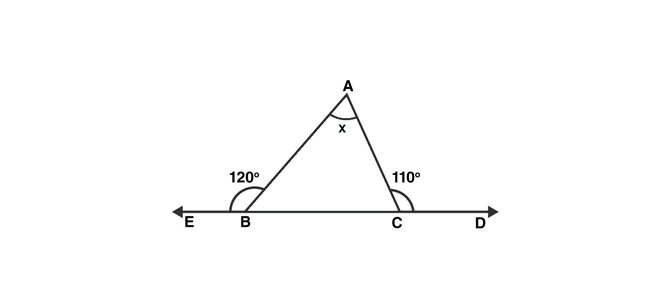Solution:

∠ABC = 180° – 120° = 60° [Linear pair]

∠ACB = 180° – 110° = 70° [Linear pair]

Sum of all angles of a triangle = 180°

x = ∠BAC = 180° − ∠ABC − ∠ACB

= 180° – 60° – 70° = 50° (ans)

### (iii)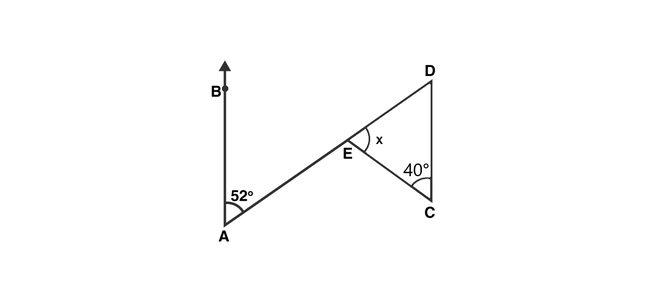Solution:

∠BAE = ∠EDC = 52° [Alternate angles]

Sum of all angles of a triangle = 180°

x = 180° – 40° – 52° = 180° − 92° = 88° (ans)

### (iv)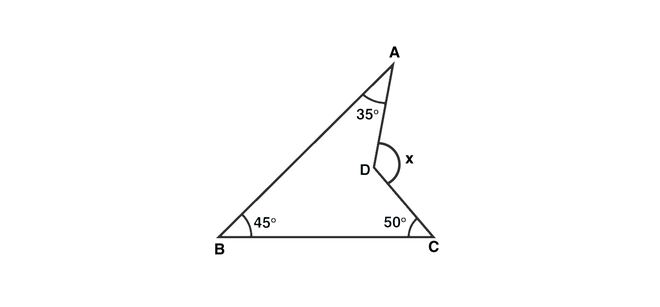Solution: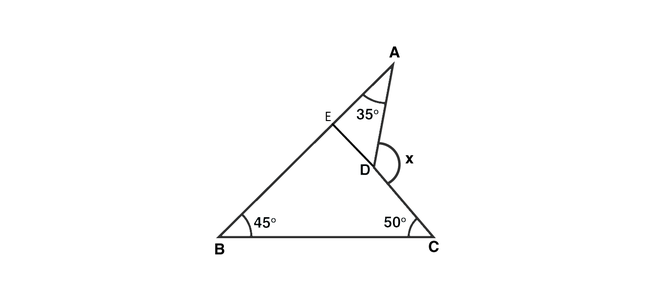CD is produced to meet AB at E.

∠BEC = 180° – 45° – 50° = 85° [Sum of all angles of a triangle = 180°]

∠AEC = 180° – 85° = 95° [Linear Pair]

Now, x = 95° + 35° = 130° [Exterior angle Property]

### Question 5: In thefigure, AB divides ∠DAC in the ratio 1 : 3 and AB = DB. Determine the value of x.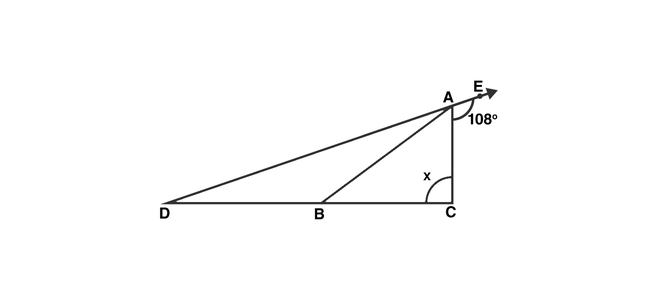Solution:

Let ∠BAD = y, ∠BAC = 3y

∠BDA = ∠BAD = y (As AB = DB)

Now,

∠BAD + ∠BAC + 108° = 180° [Linear Pair]

y + 3y + 108° = 180°

4y = 72°

or y = 18°

∠ADC + ∠ACD = 108° [Exterior Angle Property]

x + 18° = 180°

x = 90° (ans)

### Question 6: ABC is a triangle. The bisector of the exterior angle B and the bisector of ∠C intersect each other at D. Prove that ∠D = (1/2)∠A.

Solution: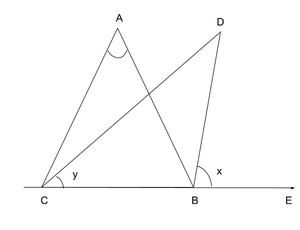Let ∠ABE = 2x and ∠ACB = 2y

∠ABC = 180° – 2K [Linear pair]

∠A = 180° — ∠ABC — ∠ACB [Angle sum property]

= 180° -180° + 2x – 2y

= 2(x – y)

Now, ∠D = 180° – ∠DBC – ∠DCB

∠D = 180° -(x + 180° – 2x) – y

= x – y

= (1/2)∠A (Hence Proved)

### Question 7: In the figure, AC is perpendicular to CE and ∠A:∠B:∠C =  3:2:1 Find ∠ECD.Solution:

Given that ∠A:∠B:∠C = 3:2:1

Let the angles be 3x, 2x and x.

3x + 2x + x = 180° [Angle Sum property]

6x = 180°

x = 30° = ∠ACB

Therefore,

∠ECD = 180° – ∠ACB – 90° [Linear Pair]

= 180° – 30° – 90°

= 60° (ans)

### Question 8: In the figure, AM is perpendicular to BC and AN is thebisector of ∠A. If ∠B = 65° and ∠C = 33° find ∠MAN.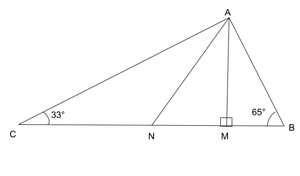Solution:

Let ∠BAN = ∠NAC = x [AN bisects ∠A]

Therefore, ∠ANM = x + 33° [Exterior angle property]

In △AMB,

∠BAM = 90° – 65° = 25° [Exterior angle property]

Therefore, ∠MAN = ∠BAN – ∠BAM = x – 25°

Now in △MAN,

(x – 25°) + (x + 33°) + 90° = 180° [Angle sum property]

or, 2x + 8° = 90°

or x = 41°

Therefore, ∠MAN = 41° – 25° = 16° (ans)

### Question 9: In a △ABC, AD bisects ∠A and ∠C > ∠B. Prove that ∠ADB > ∠ADC.

Solution: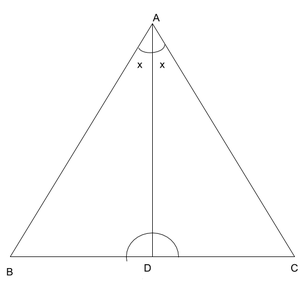Given that,

∠C > ∠B

or, ∠C + x > ∠B + x [Adding x on both sides]

### Question 10: In a △ABC, BD is perpendicular to AC and CE is perpendicular to AB. If BD and CE intersect at O prove that ∠BOC = 180° -∠A.

Solution: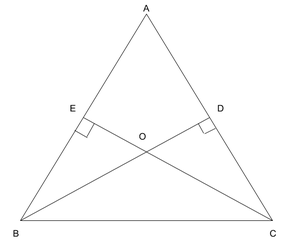∠A + ∠AEO + ∠EOD + ∠ADO = 360°

or, ∠A + 90° + 90° + ∠EOD = 360°

or, ∠A + ∠BOC = 360° – 90° – 90° [∠EOD = ∠BOC as they are vertically opposite angles]

or, ∠BOC = 180° – ∠A (proved)

### Question 11: In the figure, AE bisects ∠CAD and ∠B = ∠C. Prove that AE || BC.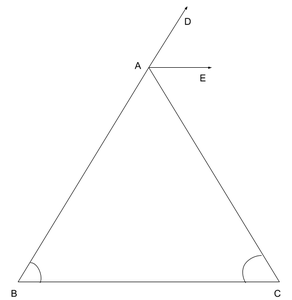Solution:

Let ∠B = ∠C = x

Then,

∠CAD = ∠B + ∠C = 2x (Exterior Angle)

∠EAC = ∠C [AE bisects ∠CAD and ∠C = x assumed]

These are interior angles for line AE and BC,

Therefore,

AE || BC (proved)

### Question 12: In the figure, AB || DE. Find ∠ACD.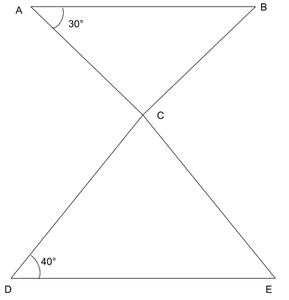Solution:

Since, AB || DE

Therefore,

∠ABC = ∠CDE = 40° [Alternate Angles]

∠ACB = 180° – ∠ABC – ∠BAC

= 180° – 40° – 30°

= 110°

Therefore,

∠ACD = 180° – ∠ACB [Linear Pair]

=70°

### Question 13. Which of the following statements are true (T) and which are false (F) :

(i) Sum of the three angles of a triangle is 180°.

(ii) A triangle can have two right angles.

(iii) All the angles of a triangle can be less than 60°.

(iv) All the angles of a triangle can be greater than 60°.

(v) All the angles of a triangle can be equal to 60°.

(vi) A triangle can have two obtuse angles.

(vii) A triangle can have at most one obtuse angles.

(viii) If one angle of a triangle is obtuse, then it cannot be a right-angled triangle.

(lx) An exterior angle of a triangle is less than either of its interior opposite angles.

(x) An exterior angle of a triangle is equal to the sum of the two interior opposite angles.

(xi) An exterior angle of a triangle is greater than the opposite interior angles.

### Question 14: Fill in the blanks to make the following statements true

(i) Sum of the angles of a triangle is _________.

(ii) An exterior angle of a triangle is equal to the two ____________ opposite angles.

(iii) An exterior angle of a triangle is always _________________ than either of the interior opposite angles.